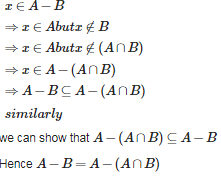# Maths Sets - Online Test

Q1. If A and B are two sets, then {tex}A \cap (A \cup B)'{/tex} is equal to
Explaination / Solution:

{tex}A \cap (A \cup B)' = \phi {/tex} since {tex}(A \cup B)'{/tex} Set represent the element which are not belongs to it,so there is no common element with the set A.So the answer is null set
Q2. Let A and B be two sets such that {tex}n(A)=35,n(B)=42\quad and\quad n(A\cap B)=17{/tex}, find {tex}n(A-B){/tex}
Explaination / Solution:
No Explaination.

Q3. If A is any set , then
Explaination / Solution:

{tex}A \cup A' = \{ x \in U:x \in A\} \cup \{ x \in U:x \notin A\} = U{/tex}

Q4. If A and B be any two sets , then (A∪BA∪B)′ is equal to
Explaination / Solution: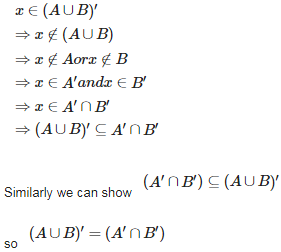Q5. If A and B be any two sets , then (A∩B) ‘ is equal to
Explaination / Solution: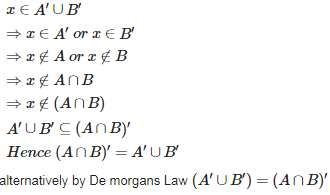Q6. Let A = { 1,2,3,4} , B = { 2,3,4,5,6 } , then (A∩B)(A∩B)is equal to :
Explaination / Solution: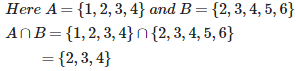Q7. Let A = { a,b,c } , B = { b,c,d } , C = { a,b,d,e } , then A∩(B∪C) is
Explaination / Solution: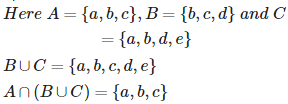Q8. Let U = { 1,2,3,4,5,6,7,8,9,10 } , A = { 1,2,5 } , B = { 6,7 }. Then A∩B′ is
Explaination / Solution: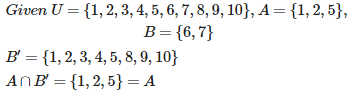Q9. If A and B are two sets , then A∪B=A∩BA∪⁡B=A∩⁡B iff
Explaination / Solution: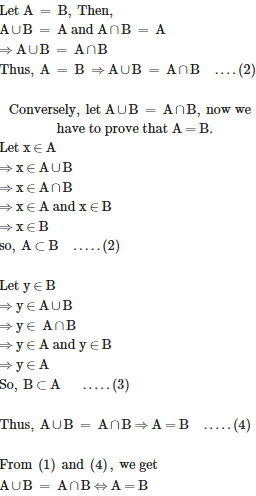Q10. Let A and B be two sets in the universal set . Then A - B is equal to
Explaination / Solution: